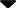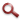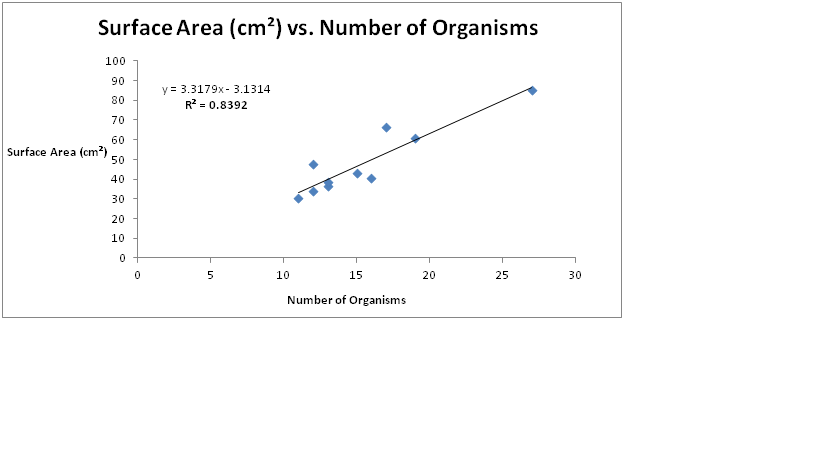Site WebYou are here:

## Student Project FiguresFigure 11 Surface Area (cm²) vs. Number of Organisms. (N=22) Data points (not jittered) represent number of organism counted on each ascidian. Line represents linearity and R² value represents Pearson Correlation Coefficient. A two-sided t-test revealed significant (p<0.05) effect of surface area on number of organisms (t = 10.989, df = 43, p-value = P<0.0001). Figure 11 Figure 12